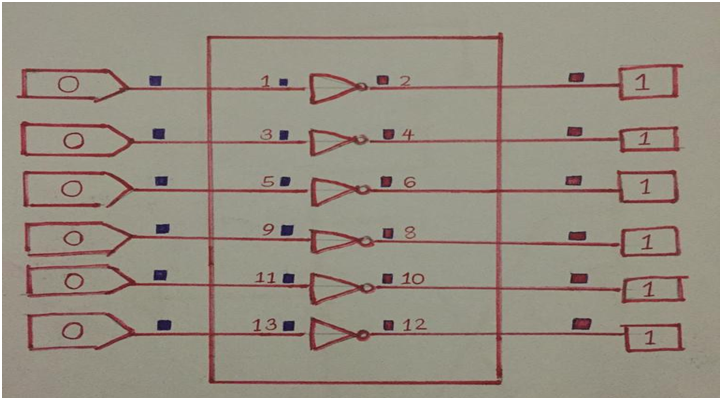Blog  /  Hex Inverter IC: All You Need to Know

# Hex Inverter IC: All You Need to Know

As an integrated circuit, Hex inverter IC (hex is short for Hexa; meaning six) has numerous purposes.

They include adding a delay, shifting levels, acting as converters between logic families, and acting as analog amplifiers.

But what exactly are hex drivers functions and how do they work, what makes them a critical component in digital devices.

In addition, what are some of the features of a hex inverter IC? This article answers all the above questions and more you may have concerning a hex inverter IC.

## Hex Drivers by Function.

Below, we look at the various hex drivers and their roles.

4069- consists of six inverter circuits. You may use this CMOS hex inverter in all applications that do not need the capabilities of a logic-level converter and medium power TTL drive.

7414- Has Schmit trigger (LSSTL) inputs.

Consequently, the Schmitt trigger inputs convert slow-changing input signals into well-defined output signals.

7407- This is a hex buffer constituting open collector outputs. The hex buffer IC has three-stage internal circuits, enabling a stable output and noise immunity.

7405- Made up of high voltage output and an open collector; hence you can guess from its name that it provides a high voltage output.

7404- Also called a Buffer and a 14-dip variation IC (integrated circuit). Thus the six inverters enable insertion action.

## Hex Inverter IC: 74HCT04

The 74HCT04 inverter IC comprises six self-sustaining inverters with push and pulls outputs.

Pins on a 7404hex inverter IC are low-powered Schottky making it a high-speed IC.

Moreover, the IC has a diode network protection system that safeguards input pins against excessive input Vcc.

The diodes can do so by activating current restraining resistors.

### Pin Configuration

First, let us look at the pin configuration of the 74HCT04 Hex inverter IC.

74HCT04 Pin Diagram

Please note that the 74HCT04 contains NOT Gates in every chip.

### Features

•  Has a voltage of range 4.5V to 5.5V
•  Completely Lead-free and RoHS certified
•  Obtainable in TSSOP -14 and SO- 14 packages
•  +25Ma continuous output current
•  2V Minimum level high input voltage
•  0.8V maximum input low-level voltage
•  TTL compatible pins
•  29 ns Transition time
•  Input pins possess Schmitt trigger action.
•  Six self-standing inverters (NOT Gate )IC

### Applications

The 74HCT04 Hex inverter IC has many applications. They include

• It may be used in the generation of clock signals.
•  Square wave generator.
•  It can serve as a buffer.
•  Interface NMOS and TTL outputs.

### How to use 74HCT04 hex inverter IC

Using the 74HCTO4 is not complex, as you may have noticed that all the six NOT Gates have their input and output pins.

Consequently, all you have to do is link pin 16 to the positive supply voltage and pin 8 to the circuit's ground, and you are done.

However, to fully understand how the IC works, we must first look at a NOT Gates behavior when linked with an input signal.

For example, we can say the input signal is F, and the output signal is G. Hence this NOT Gates Boolean equation would be G=~F.

If the output voltage is high, the input voltage will be low and vice versa. Thus the NOT Gates truth diagram would be as shown in the chart below.

Note that all the 74HCT04 integrated circuits, NOT Gates, will produce a similar truth diagram like the one above when tested.

### Example Circuit.

Now that you have an idea of how the NOT Gates work. Let us look at some circuits examples which use a 74HCT04 hex inverter.

#### 74HCT04 inverter circuit Propagation delay

Switching time, also called propagation delay, refers to the duration the output pin takes to convert from one state to another.

The 74HCT04 has a 29-nanosecond propagation delay, making it perfect for numerous applications like the ones stated earlier.

Below is a 7404 IC switching diagram to better understand.

74HCT04 Switching diagram

.74HCT04 circuit diagram.

After viewing the above 74HCT04 circuit diagram, you will realize that it utilizes all the hex IC inverters.

In addition, all the inverters show the same truth diagram; when the input is zero, the output will be one, and the same is true in reverse.

## Hex Inverter IC: CD4069

The hex inverter CD4069 works similarly to the 74HCT04. They even share some features such as noise immunity and consistently managed rise and fall durations.

Meaning, you can use the CD4069 for many inverter applications, such as reasonable conversion levels of circuits.

### CD4069 Pinout

CD4069 pinout diagram

Source: Wikipedia

### Illustrative/ Schematic Diagram

Illustrative/ Schematic Diagram

You can see from the above diagram that the CD4069 inverter gates have a MOSFET P and N structure.

The MOSFET design thus goes to show that the CD4069 provides a nice inverter gate logic utilizing low energy.

### Applications

• Making oscillators
• Designing Amplifiers with the high input impedance
• Pulse framing
• Logic reversal

### Example Circuit

The ricochet switch circuit diagram below showcases a perfect example of the CD4069 functioning in a circuit.

CD4069 circuit diagram

CD4069 application Circuit

Where

R1 and R2 are resistors, and the CD4069 inverter works by inverting the 3V input into 16V output.

Please note that when you connect all of them to VSS (GND), you protect your inputs from static electricity.

correct VSS(GND) connection

## Summary

After going through this article, you should easily state the various circuit applications of a hex inverter IC. Explain the driver functions and understand how a Not Gate works.

In conclusion, if you require clarification on these matters, do not hesitate to contact us.